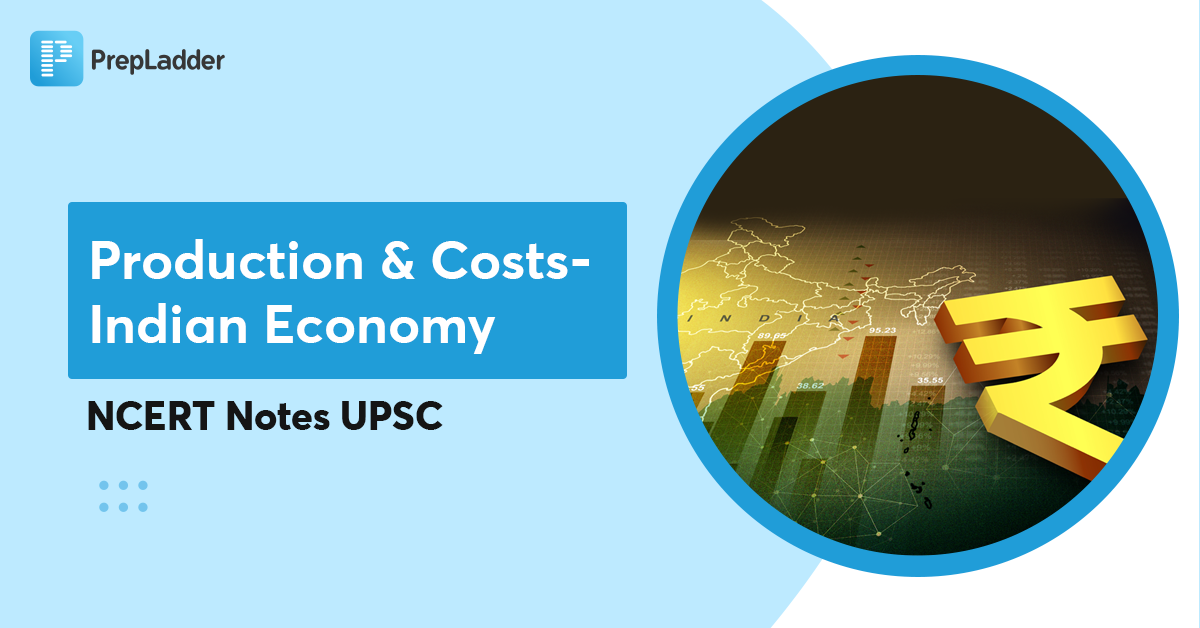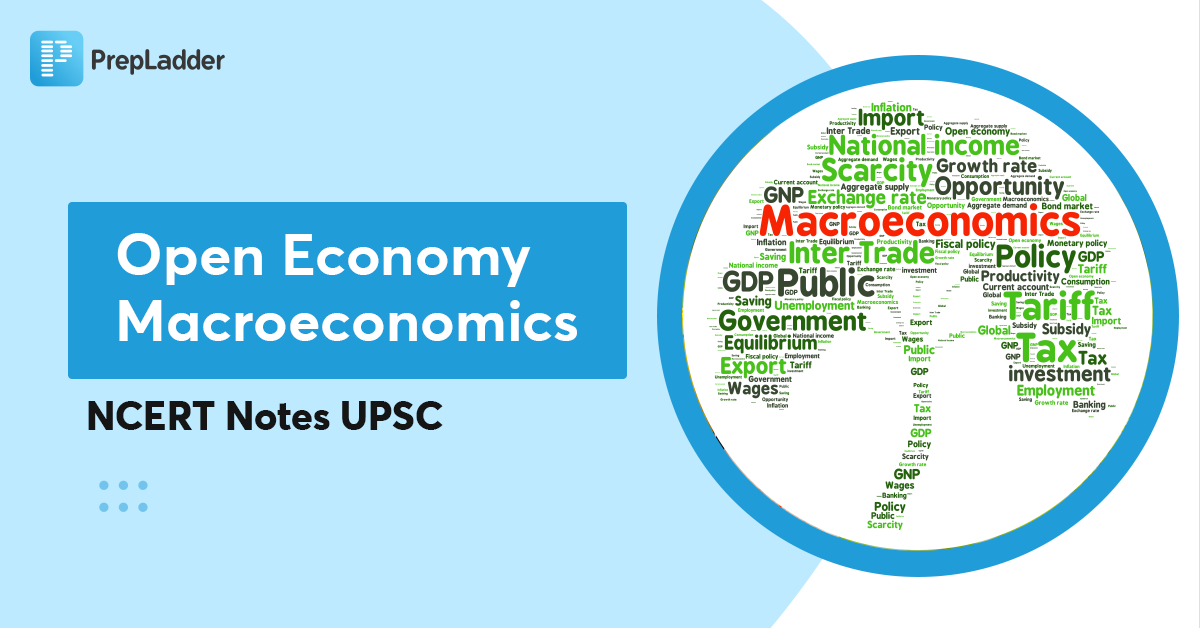## Production and Costs- Indian Economy - NCERT Notes UPSCProduction is the process by which inputs are transformed into ‘output’. Production is carried out by producers or firms. A firm uses different factors of production (inputs) such as land, labour, machines, raw materials etc. to produce output. This output can be consumed by consumers or used by other firms for further production.

However, to acquire inputs a firm has to pay for them. This is called the cost of production. Once output has been produced, the firm sell it in the market and earns revenue. The difference between the revenue and cost is called the firm’s profit.

Navigate through the blog to understand the topic in detail and enhance your UPSC CSE preparation

## Production Function

• It is a relationship between inputs used and output produced by the firm.
• It gives an idea about the maximum quantity of output that can be produced for various quantities of inputs used.
• It is defined for a given technology. If the technology improves, the maximum levels of output obtainable for different input combinations increase. We then have a new production function.

## Time concepts in Production

• Short Run: In short run, at least one of the factors: labour or capital – cannot be varied, and therefore, remains fixed. Therefore, to vary the output level, the firm can vary only the other factor. The factor that remains fixed is called the fixed factor whereas the other factor which the firm can vary is called the variable factor.
• Long Run: All factors of production can be varied, due to the long time frame being considered. There is no fixed factor in this concept.

Also, watch a related video on The Macroeconomics Parameters by Vivek Singh Sir, our Economy faculty, and upgrade your UPSC Mains 2022 preparation:

## Other Important Terms related to Production

Total Product (TP): It is a relationship between the variable input and output.  Total product is the output received at a particular level of the Variable Input, keeping all other factors constant

• Average product (AP): It is defined as the output per unit of variable input.
• Marginal product of an input (MP): It is defined as the change in output per unit of change in the input when all other inputs are held constant. For any level of an input, the sum of marginal products of every preceding unit of that input gives the total product.
• Law of Variable Proportions: It is defined as Marginal Product of a variable factor input initially rises with its input level but after reaching a certain level of employment, it starts falling.

Curve (TP, AP and MP)

Beyond a certain point, the production process becomes too crowded with the variable input and further effectiveness can only be attained by enhancing the fixed input.  However, this is a Short Run concept as in the Long Run all the factors are Variable.

## Returns to Scale

• It is the quantitative change in output of a firm resulting from a proportionate increase in all inputs.
• When a proportional increase in all inputs results in an increase in output by the same proportion, the production function shows a Constant Return to Scale (CRS)
• When a proportional increase in all inputs results in an increase in output by a larger proportion, the production function is said to display Increasing Returns to Scale (IRS)
• Decreasing Returns to Scale (DRS) holds when a proportional increase in all inputs results in an increase in output by a smaller proportion.

Important links for UPSC Mains preparation:

## Costs

Cost is the monetary value of all the expenditures for raw materials, equipment, labours, etc. required to produce goods and services. For every level of output, the firm chooses the least cost input combination in order to achieve price competitiveness and to maximise profit.

Thus, the cost function describes the least cost of producing each level of output given prices of factors of production and technology.

• Total Fixed Cost: In the short run, some of the factors of production cannot be varied, and therefore, remain fixed. The cost that a firm incurs to employ fixed inputs is called the total fixed cost.
• Total Variable Cost: To produce any required level of output, the firm, in the short run, can adjust only variable inputs. Accordingly, the cost that a firm incurs to employ these variable inputs is called the total variable cost (TVC).
• Short Run Average Cost: It is defined as the total cost per unit of output. At zero output, short run average cost is undefined.
• Short Run Marginal Cost: It is defined as the change in total cost per unit of change in output.
• Average Variable Cost: It is defined as the total variable cost per unit of output in short term production. If the price of a good is higher than the average variable cost of the good, the firm is covering all the variable costs and a percentage of the fixed costs. In this case, firms continue production.## Current Affairs

•••••••••••••••